# Bi-unitary multiperfect numbers, IV(a)

Pentti Haukkanen and Varanasi Sitaramaiah
Notes on Number Theory and Discrete Mathematics
Print ISSN 1310–5132, Online ISSN 2367–8275
Volume 26, 2020, Number 4, Pages 2—32
DOI: 10.7546/nntdm.2020.26.4.2-32

## Details

### Authors and affiliations

Pentti HaukkanenFaculty of Information Technology and Communication Sciences
FI-33014 Tampere University, Finland

Varanasi Sitaramaiah
1/194e, Poola Subbaiah Street, Taluk Office Road, Markapur
Prakasam District, Andhra Pradesh, 523316 India

### Abstract

A divisor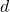of a positive integer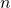is called a unitary divisor if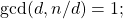andis called a bi-unitary divisor ofif the greatest common unitary divisor ofand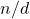is unity. The concept of a bi-unitary divisor is due to D. Surynarayana (1972). Letdenote the sum of the bi-unitary divisors of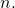A positive integeris called a bi-unitary multiperfect number iffor some. For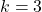we obtain the bi-unitary triperfect numbers.

Peter Hagis (1987) proved that there are no odd bi-unitary multiperfect numbers. The present paper is Part IV(a) in a series of papers on even bi-unitary multiperfect numbers. In parts I, II and III we found all bi-unitary triperfect numbers of the form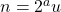, where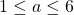andis odd. There exist exactly ten such numbers. In this part we solve partly the case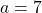. We prove that ifis a bi-unitary triperfect number of the form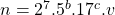, where, then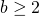. We then confine ourselves to the case. We prove that in this case we haveand further show that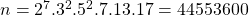is the only bi-unitary triperfect number of this form.

### Keywords

• Perfect numbers
• Triperfect numbers
• Multiperfect numbers
• Bi-unitary analogues

• 11A25

### References

1. Hagis, P., Jr. (1987). Bi-unitary amicable and multiperfect numbers, Fibonacci Quart., 25 (2), 144–150.
2. Haukkanen, P. & Sitaramaiah, V. (2020). Bi-unitary multiperfect numbers, I, Notes Number Theory Discrete Math., 26 (1), 93–171.
3. Haukkanen, P., & Sitaramaiah, V. (2020). Bi-unitary multiperfect numbers, II. Notes on Number Theory and Discrete Mathematics, 26 (2), 1-26.
4. Haukkanen, P., & Sitaramaiah, V. (2020). Bi-unitary multiperfect numbers, III. Notes on Number Theory and Discrete Mathematics, 26 (3), 33-67.
5. Sándor, J. & Crstici, P. (2004). Handbook of Number Theory II, Kluwer Academic.
6. Suryanarayana, D. (1972). The number of bi-unitary divisors of an integer, in The Theory of Arithmetic Functions, Lecture Notes in Mathematics 251: 273–282, New York, Springer–Verlag.
7. Wall, C. R. (1972). Bi-unitary perfect numbers, Proc. Amer. Math. Soc., 33, No. 1, 39–42.

## Cite this paper

Haukkanen, P., & Sitaramaiah, V. (2020). Bi-unitary multiperfect numbers, IV(a). Notes on Number Theory and Discrete Mathematics, 26(4), 2-32, doi: 10.7546/nntdm.2020.26.4.2-32.